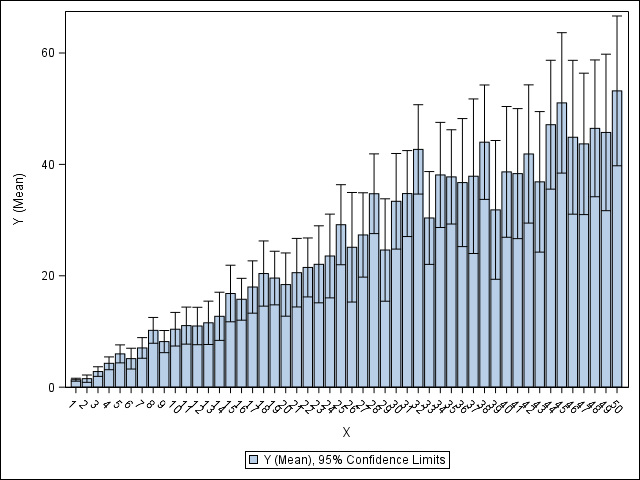## SGPLOT VBAR XAXIS Label Interval?

SGPLOT VBAR is drawing all the 50 bars needed, but can I just apply label intervals to the x-axis below so that it changes from 1, 2, 3, …, 48, 49, 50 to 5, 10, 15, …, 40, 45, 50, for example?

``````data SAMPLE;
call streaminit(1);
do X=1 to 50;
do ITERATION=1 to 50;
Y=rand('normal',X,X);
output;
end;
end;
run;

ods results=off;
ods listing gpath='!userprofile\desktop\';
ods graphics/reset imagename='SGPLOT';

proc sgplot;
vbar X/response=Y stat=mean limits=both;
run;``````1 ACCEPTED SOLUTION

Accepted Solutions

## Re: SGPLOT VBAR XAXIS Label Interval?

Hello @Junyong,

First of all, thank you very much for providing readily usable example data and code.

I think adding this XAXIS statement to your PROC SGPLOT step will help:

``xaxis values=(5 to 50 by 5) type=linear valueshint;``

The default axis type in your case would be DISCRETE, not LINEAR, so that the VALUES= specification alone would also restrict the set of bars drawn. Without the VALUESHINT option SAS would not leave enough space for the bars with X values less than the first tick mark value (5).

## Re: SGPLOT VBAR XAXIS Label Interval?

Hello @Junyong,

First of all, thank you very much for providing readily usable example data and code.

I think adding this XAXIS statement to your PROC SGPLOT step will help:

``xaxis values=(5 to 50 by 5) type=linear valueshint;``

The default axis type in your case would be DISCRETE, not LINEAR, so that the VALUES= specification alone would also restrict the set of bars drawn. Without the VALUESHINT option SAS would not leave enough space for the bars with X values less than the first tick mark value (5).

Discussion stats